Processing ......FreeComputerBooks.com Links to Free Computer, Mathematics, Technical Books all over the World

Basic Category Theory
🌠 Top Free Computer Networking Books - 100% Free or Open Source!
• Title Basic Category Theory
• Author(s) Tom Leinster
• Publisher: Cambridge University Press; 1 edition (September 22, 2014); eBook (arxiv.org)
• Hardcover 190 pages
• eBook PDF
• Language: English
• ISBN-10/ASIN: 1107044243
• ISBN-13: 978-1107044241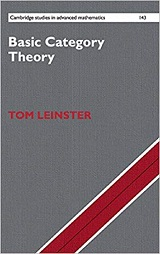Book Description

Assuming little mathematical background, this short introduction to Category Theory is ideal for beginning graduate students or advanced undergraduates learning category theory for the first time. Suitable for independent study or as a course book, it gives extensive explanations of the key concepts along with hundreds of examples and exercises.

At the heart of this short introduction to category theory is the idea of a universal property, important throughout mathematics. After an introductory chapter giving the basic definitions, separate chapters explain three ways of expressing universal properties: via adjoint functors, representable functors, and limits. A final chapter ties all three together.

The book is suitable for use in courses or for independent study. Assuming relatively little mathematical background, it is ideal for beginning graduate students or advanced undergraduates learning category theory for the first time.

For each new categorical concept, a generous supply of examples is provided, taken from different parts of mathematics. At points where the leap in abstraction is particularly great (such as the Yoneda lemma), the reader will find careful and extensive explanations. Copious exercises are included.

• Tom Leinster has held postdoctoral positions at Cambridge and the Institut des Hautes Études Scientifiques (France), and held an EPSRC Advanced Research Fellowship at the University of Glasgow. He is currently a Chancellor's Fellow at the University of Edinburgh.
Reviews, Rating, and Recommendations: Related Book Categories: Read and Download Links:Similar Books:
•An Invitation to Applied Category Theory: Seven Sketches

Category theory is now a powerful tool in science, informatics, and industry. This book offers a self-contained tour of applied category theory. Each chapter follows a single thread motivated by a real-world application and discussed with category-theoretic tools.

•Topology: A Categorical Approach (Tai-Danae Bradley, et al)

A graduate-level textbook that presents basic topology from the perspective of category theory. Many graduate students are familiar with the ideas of point-set topology and they are ready to learn something new about them.

•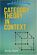Category Theory in Context (Emily Riehl)

This book introduces the essential concepts of category theory: categories, functors, natural transformations, the Yoneda lemma, limits and colimits, adjunctions, monads, Kan extensions, and other topics.

•Category Theory for the Sciences (David I. Spivak)

Using databases as an entry to Category Theory, this book explains category theory by examples, and shows that category theory can be useful outside of mathematics as a rigorous, flexible, and coherent modeling language throughout the sciences.

•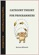Category Theory for Programmers (Bartosz Milewski)

In this category theory for programmers, the author illustrates all major concepts using computer code. You are probably aware that functional languages are closer to math than the more popular imperative languages. They also offer more abstracting power.

•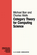Category Theory for Computing Science (Michael Barr, et al.)

This book is a textbook in basic category theory, written specifically to be read by people in computing science. It expounds the constructions we feel are basic to category theory in the context of examples and applications to computing science.

•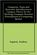Categories, Types, and Structures (Andrea Asperti, et al)

This book introduces Category Theory at a level appropriate for computer scientists and provides practical examples in the context of programming language design. It pursues the more complex mathematical semantics of data types and programs.

•Categorical Homotopy Theory (Emily Riehl)

This book develops abstract homotopy theory from the categorical perspective with a particular focus on examples. It helps consolidate and simplify one's understanding of derived functors, homotopy limits and colimits, and model categories, among others.

•Higher Topos Theory (Jacob Lurie)

This book presents the foundations of Higher Topos Theory, using the language of weak Kan complexes, and shows how existing theorems in algebraic topology can be reformulated and generalized in the theory's new language.

•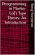Programming in Martin-Lof's Type Theory: An Introduction

This book focuses on the type theory developed by Per Martin-Lof. It contains a thorough introduction to the Martin-Lof's Type Theory, with information on polymorphic sets, subsets, monomorphic sets, and a full set of helpful examples.

Book Categories
 :All CategoriesTop Free BooksRecent BooksMiscellaneous BooksComputer EngineeringComputer LanguagesComputer ScienceData Science/DatabasesJava and Java EE (J2EE)Linux and UnixMathematicsMicrosoft and .NETMobile ComputingNetworking and CommunicationsSoftware EngineeringSpecial TopicsWeb Programming
Other Categories